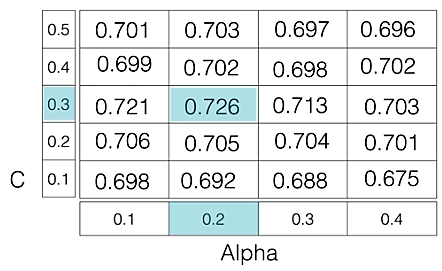# ML | Hyperparameter tuning

A Machine Learning model is defined as a mathematical model with a number of parameters that need to be learned from the data. By training a model with existing data, we are able to fit the model parameters.
However, there is another kind of parameters, known as Hyperparameters, that cannot be directly learned from the regular training process. They are usually fixed before the actual training process begins. These parameters express important properties of the model such as its complexity or how fast it should learn.

Some examples of model hyperparameters include:

1. The penalty in Logistic Regression Classifier i.e. L1 or L2 regularization
2. The learning rate for training a neural network.
3. The C and sigma hyperparameters for support vector machines.
4. The k in k-nearest neighbors.

Models can have many hyperparameters and finding the best combination of parameters can be treated as a search problem. Two best strategies for Hyperparameter tuning are:

GridSearchCV
In GridSearchCV approach, machine learning model is evaluated for a range of hyperparameter values. This approach is called `GridSearchCV`, because it searches for best set of hyperparameters from a grid of hyperparameters values.

For example, if we want to set two hyperparameters C and Alpha of Logistic Regression Classifier model, with different set of values. The gridsearch technique will construct many versions of the model with all possible combinations of hyerparameters, and will return the best one.

As in the image, for C = [0.1, 0.2, 0.3, 0.4, 0.5] and Alpha = [0.1, 0.2, 0.3, 0.4].
For a combination C=0.3 and Alpha=0.2, performance score comes out to be 0.726(Highest), therefore it is selected.Following code illustrates how to use GridSearchCV

 `# Necessary imports ` `from` `sklearn.linear_model ``import` `LogisticRegression ` `from` `sklearn.model_selection ``import` `GridSearchCV ` ` `  `# Creating the hyperparameter grid ` `c_space ``=` `np.logspace(``-``5``, ``8``, ``15``) ` `param_grid ``=` `{``'C'``: c_space} ` ` `  `# Instantiating logistic regression classifier ` `logreg ``=` `LogisticRegression() ` ` `  `# Instantiating the GridSearchCV object ` `logreg_cv ``=` `GridSearchCV(logreg, param_grid, cv ``=` `5``) ` ` `  `logreg_cv.fit(X, y) ` ` `  `# Print the tuned parameters and score ` `print``(``"Tuned Logistic Regression Parameters: {}"``.``format``(logreg_cv.best_params_))  ` `print``(``"Best score is {}"``.``format``(logreg_cv.best_score_)) `

Output:

Tuned Logistic Regression Parameters: {‘C’: 3.7275937203149381}
Best score is 0.7708333333333334

Drawback : GridSearchCV will go through all the intermediate combinations of hyperparameters which makes grid search computationally very expensive.

RandomizedSearchCV
RandomizedSearchCV solves the drawbacks of GridSearchCV, as it goes through only a fixed number of hyperparameter settings. It moves within the grid in random fashion to find the best set hyperparameters. This approach reduces unnecessary computation.

Following code illustrates how to use RandomizedSearchCV

 `# Necessary imports ` `from` `scipy.stats ``import` `randint ` `from` `sklearn.tree ``import` `DecisionTreeClassifier ` `from` `sklearn.model_selection ``import` `RandomizedSearchCV ` ` `  `# Creating the hyperparameter grid  ` `param_dist ``=` `{``"max_depth"``: [``3``, ``None``], ` `              ``"max_features"``: randint(``1``, ``9``), ` `              ``"min_samples_leaf"``: randint(``1``, ``9``), ` `              ``"criterion"``: [``"gini"``, ``"entropy"``]} ` ` `  `# Instantiating Decision Tree classifier ` `tree ``=` `DecisionTreeClassifier() ` ` `  `# Instantiating RandomizedSearchCV object ` `tree_cv ``=` `RandomizedSearchCV(tree, param_dist, cv ``=` `5``) ` ` `  `tree_cv.fit(X, y) ` ` `  `# Print the tuned parameters and score ` `print``(``"Tuned Decision Tree Parameters: {}"``.``format``(tree_cv.best_params_)) ` `print``(``"Best score is {}"``.``format``(tree_cv.best_score_)) `

Output:

Tuned Decision Tree Parameters: {‘min_samples_leaf’: 5, ‘max_depth’: 3, ‘max_features’: 5, ‘criterion’: ‘gini’}
Best score is 0.7265625

My Personal Notes arrow_drop_upCheck out this Author's contributed articles.

If you like GeeksforGeeks and would like to contribute, you can also write an article using contribute.geeksforgeeks.org or mail your article to contribute@geeksforgeeks.org. See your article appearing on the GeeksforGeeks main page and help other Geeks.

Please Improve this article if you find anything incorrect by clicking on the "Improve Article" button below.

Article Tags :
Practice Tags :

Be the First to upvote.

Please write to us at contribute@geeksforgeeks.org to report any issue with the above content.